# Mathematical problems for self assessment

###### FIRST ORDER ORDINARY DIFFERENTIAL EQUATIONS

1. For the following differential equations, state their order and whether they are linear or nonlinear equations:

(i)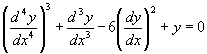(ii)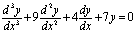(iii)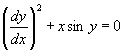(iv)[Ans. (i) order 4, nonlinear, (ii) order 3, linear, (iii) order 1, nonlinear, (iv) order 1, linear].

2. Verify that the function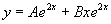solves the differential equation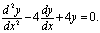3. Determine the differential equations satisfied by the following functions:

(i)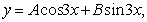(ii)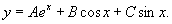[Ans. (i)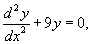(ii)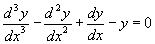].

4. Find the solutions of the following differential equations by direct integration:

(i)(ii)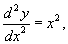,

(iii)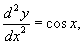[Ans. (i)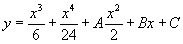, (ii)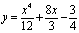, (iii)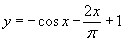].

5. Find, by separation of variables, the particular solutions of the differential equations:

(i)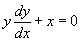, y(-2) =4,

(ii)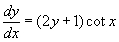,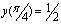.

[Ans. (i)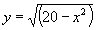, (ii)].

6. Find, by separation of variables, the general solutions of the differential equations:

(i),

(ii).

[Ans. (i)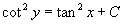, (ii)].

7. By finding an appropriate integrating factor determine the general solutions of the following linear ordinary differential equations:

(i)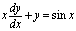,

(ii)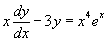.

[Ans. (i)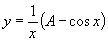, (ii)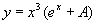].

8. By finding an appropriate integrating factor determine the particular solution of the linear ordinary differential equation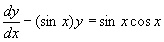,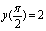.

[Ans.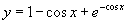].

9. In an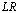circuit with applied voltage,the instantaneous current i(t) in the circuit satisfies the first order ordinary differential equation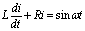with initial condition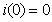. Find the particular solution of this linear ODE.

[Ans.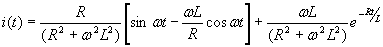].

10. (The Bernoulli Equation) Consider the nonlinear ordinary differential equation given by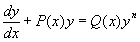, for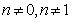and appropriate functions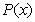and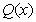. This equation is called the Bernoulli equation and it has particular applications in fluid mechanics and civil engineering problems. Show that by making the substitution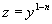, this equation can be expressed in the form,

which is a linear equation that can be solved in the standard way using the integrating factor technique.

Hence find the general solution of the Bernoulli equation.

[Ans.].